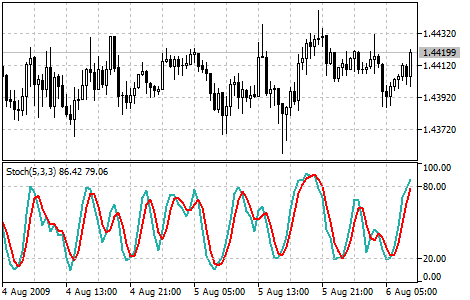# Stochastic Oscillator

The Stochastic Oscillator Technical Indicator compares where a security’s price closed relative to its price range over a given time period. The Stochastic Oscillator is displayed as two lines. The main line is called %K. The second line, called %D, is a Moving Average of %K. The %K line is usually displayed as a solid line and the %D line is usually displayed as a dotted line. There are several ways to interpret a Stochastic Oscillator. Three popular methods include:

• Buy when the Oscillator (either %K or %D) falls below a specific level (for example, 20) and then rises above that level. Sell when the Oscillator rises above a specific level (for example, 80) and then falls below that level.
• Buy when the %K line rises above the %D line and sell when the %K line falls below the %D line.
• Look for divergences. For instance: where prices are making a series of new highs and the Stochastic Oscillator is failing to surpass its previous highs.

You can test the trade signals of this indicator by creating an Expert Advisor in MQL5 Wizard.## Calculation

Four variables are used for the calculation of the Stochastic Oscillator:

• %K periods. This is the number of time periods used in the stochastic calculation.
• %K Slowing Periods. This value controls the internal smoothing of %K. A value of 1 is considered a fast stochastic; a value of 3 is considered a slow stochastic.
• %D periods. This is the number of time periods used when calculating a moving average of %K.
• %D method. The method (i.e., Exponential, Simple, Smoothed, or Weighted) that is used to calculate %D.

The formula for %K is:

%K = (CLOSE - MIN (LOW (%K))) / (MAX (HIGH (%K)) - MIN (LOW (%K))) * 100

Where:

CLOSE — today’s closing price;
MIN (LOW (%K)) — the lowest minimum in %K periods;
MAX (HIGH (%K)) — the highest maximum in %K periods.

The %D moving average is calculated according to the formula:

%D = SMA (%K, N)

Where:

N — smoothing period;
SMA — Simple Moving Average.